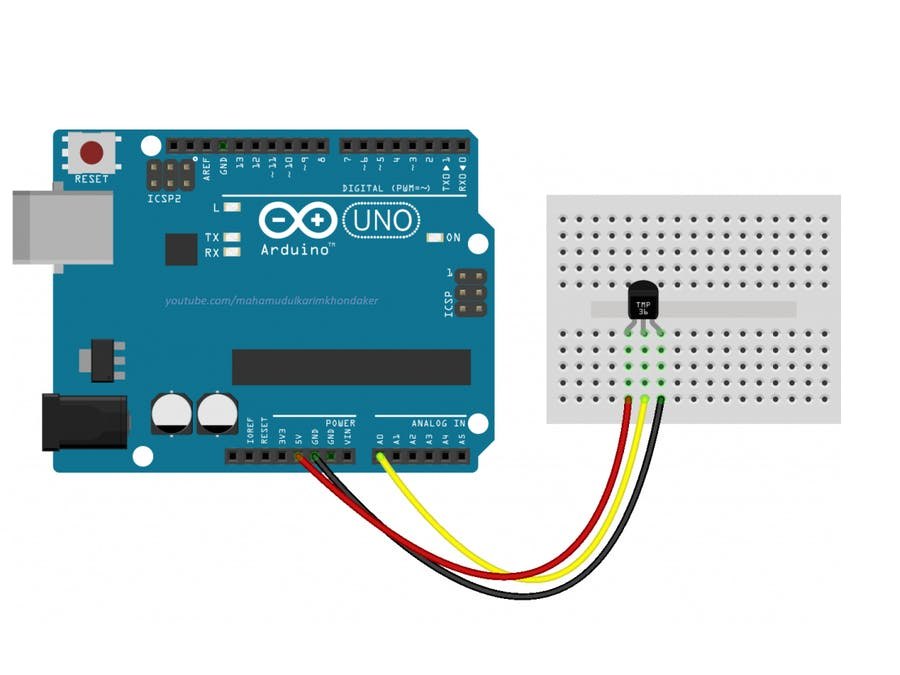# Sensors

A) Potentiometer

B) TMP36 or LM35 Temperature sensors

TMP36 sensor’s output voltage is almost linear at 10 mV/°C for a temperature range of –40 to +125°C. The accuracy is only ±2°C over the temperature range. The temperature in °C is simply calculated from Vout using the formula:
T = 100Vout – 50
The constant 50 is specified in the data sheet for the TMP36. If you are using LM35 sensor there is no offset value of 50. In Arduino IDE choose Serial monitor and Serial plot to see the values or graph.``````
int sensePin = A0; //This is the Arduino Pin that will read the sensor output
int sensorInput; //The variable we will use to store the sensor input
double temp; //The variable we will use to store temperature in degrees.

void setup() {
// put your setup code here, to run once:
Serial.begin(9600); //Start the Serial Port at 9600 baud (default)
}

void loop() {
// put your main code here, to run repeatedly:
sensorInput = analogRead(A0); //read the analog sensor and store it
temp = (double)sensorInput / 1024; //find percentage of input reading
temp = temp * 5; //multiply by 5V to get voltage
temp = temp – 0.5; //Subtract the offset

temp = temp * 100; //Convert to degrees
Serial.print("Current Temperature: ");
Serial.println(temp);
}``````

C) NTC Sensor Temperature

D) DHT22 temperature and humidity sensor

E) PIR sensor

F) Ultrasound distance sensor